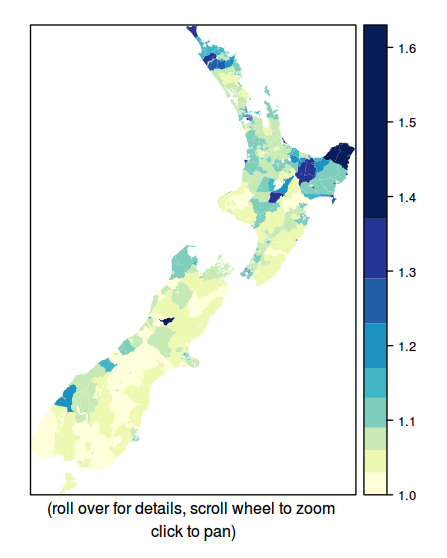# Seeing Through grid Graphics

The University of Auckland

# Seeing Through grid Graphics

The Big Idea: Don't be a Dead End

# An Example Plot

``````library(lattice)
plot <- xyplot(mpg ~ disp | trans, data=mtcars)``````

# An Example Plot

What can I do with this plot AFTER it has been drawn ?

# Not Interested

```     xyplot(x,
data,
allow.multiple = is.null(groups) || outer,
outer = !is.null(groups),
auto.key = FALSE,
aspect = "fill",
panel = lattice.getOption("panel.xyplot"),
prepanel = NULL,
scales = list(),
strip = TRUE,
groups = NULL,
xlab,
xlim,
ylab,
ylim,
drop.unused.levels = lattice.getOption("drop.unused.levels"),
...,
```

# Allow More Drawing

``````print(plot)
grid.rect()``````

# An Example Plot

What can I do with this plot AFTER it has been drawn ?

# Remember the Drawing Context

``````print(plot)
showViewport()``````

``````print(plot)
downViewport("plot_01.panel.1.2.vp")
grid.text("Ferrari Dino", x=155, y=19.7, just="left", default="native")``````

+

# Remember What was Drawn

``````print(plot)
showGrob()``````

``````print(plot)
grid.edit("plot_01.xlab", label="Engine Displacement")``````

→

# Allow Labels and Structure

``````grid.roundrect(gp=gpar(col=NA, fill=rgb(1,0,0,.5)), name="box")
grid.text("Ferrari Dino", name="label")``````

``grid.ls()``
``````box
label``````

# Allow Labels and Structure

``````gTree(children=gList(roundrectGrob(gp=gpar(col=NA, fill=rgb(1,0,0,.5)),
name="box"),
textGrob("Ferrari Dino", name="label")),
name="boxedLabel")``````

``grid.ls()``
``````boxedLabel
box
label``````

# Provide Labels and Structure

``````print(plot)
grid.ls(viewports=TRUE)``````
``````viewport[ROOT]
rect[plot_01.background]
viewport[plot_01.toplevel.vp]
viewport[plot_01.xlab.vp]
text[plot_01.xlab]
viewport[plot_01.ylab.vp]
text[plot_01.ylab]
viewport[plot_01.figure.vp]``````

# Export Labels and Structure

R code ...

```library(gridSVG)
print(plot)
grid.export()
```

SVG code ...

```<svg>
<rect id="plot_01.background.1.1"/>
<g id="plot_01.toplevel.vp.1">
<g id="plot_01.toplevel.vp::plot_01.xlab.vp.1">
<text id="plot_01.xlab.1">
...
```

# Export Labels and Structure

The Royal Society of New Zealand: Languages in Aotearoa New Zealand

# Export Labels and Structure

R code ...

```pushViewport(viewport(name="viewport"))
grid.polygon("ID:1")
grid.polygon("ID:2")
...
```

SVG code ...

```<svg>
<g id="viewport">
<polygon id="ID:1" .../>
<polygon id="ID:2" .../>
...
```

Javascript code ...

```svgRoot = root.getElementById("viewport");
...
```

# Don't be a Dead End

The Royal Society of New Zealand: Languages in Aotearoa New Zealand (original)# Don't be a Dead End

The Royal Society of New Zealand: Languages in Aotearoa New Zealand (recycled)

# Summary

• grid
• Allow more drawing
• Record the drawing context
• Record what was drawn
• Allow labels and structure
• lattice
• Provide a naming scheme
• Provide structure
• gridSVG
• Export labels and structure

# Acknowledgements

• The plot with lots of extra drawing was adapted from Felgate, Bickler, and Murrell (2013) "Estimating parent population of pottery vessels from a sample of fragments: a case study from inter-tidal surface collections, Roviana Lagoon, Solomon Islands", Journal of Archaeological Science, 40 (2013) 1319-1328.

• The raster map plot used NASA light data from Steve Mosher and a Google Map tile (copyright 2011 Google, Map Data and copyright 2011 Tele Atlas).

• The forest plot was generated using the 'metaplot' package by Murrell, Scott, and Lu

• The map of Multilingualism in New Zealand was created by Paul Behrens for the Royal Society of New Zealand